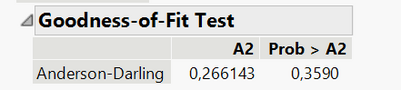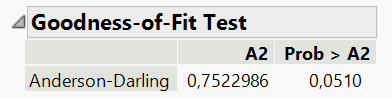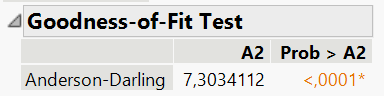Choose Language Hide Translation Bar
Highlighted

## Anderson-Darling - Shapiro Wilk

I have to test the data for normality.

First used 9 version now 15.0

As I understand from the forum the Shapiro Wilk test is outdated and the Anderson-Darling test (AD) is currently being used. AD is used to define kind of Distribution

Normal Distribution;

Cauchy;

Lognormal;

Exponential;

Gamma;

SHASH;

Weibull.

But what about a criterion. Does it look like Shapiro-Wilk's?

1.2.3.And why does Prob everytime is  > then A2 ?

1 ACCEPTED SOLUTION

Accepted Solutions
Highlighted

## Re: Anderson-Darling - Shapiro Wilk

It is a hypothesis test, so it works like the Shapiro-Wilks test. The null hypothesis is the fitted distribution. The sample test statistic is A2. It is an upper-tailed test like the chi square and F tests. A large statistics is evidence against the null. The stated probability is for A2 at least as large as the one estimated from your sample when the null hypothesis is true.

It is meant to be used to decide if you should reject the fitted model. It is not intended to be used for model selection. A criterion such as AICc would be better for that purpose.

The three examples that you provided each present a p-value for your decision. First you decide the type I error risk you are willing to take (i.e., alpha level) and then compare the p-value to alpha. If the p-value is less than alpha, you reject the null hypothesis. Otherwise, you continue to assume the null is true. For example, if I use alpha = 0.1, then I do not reject the null for the first case but I do reject the null for the other two tests.

Note that using the same hypothesis test with the same sample of the population for many different models increases the chances of type I errors. Such a practice is an example of multiple comparisons.

Learn it once, use it forever!
2 REPLIES 2
Highlighted

## Re: Anderson-Darling - Shapiro Wilk

It is a hypothesis test, so it works like the Shapiro-Wilks test. The null hypothesis is the fitted distribution. The sample test statistic is A2. It is an upper-tailed test like the chi square and F tests. A large statistics is evidence against the null. The stated probability is for A2 at least as large as the one estimated from your sample when the null hypothesis is true.

It is meant to be used to decide if you should reject the fitted model. It is not intended to be used for model selection. A criterion such as AICc would be better for that purpose.

The three examples that you provided each present a p-value for your decision. First you decide the type I error risk you are willing to take (i.e., alpha level) and then compare the p-value to alpha. If the p-value is less than alpha, you reject the null hypothesis. Otherwise, you continue to assume the null is true. For example, if I use alpha = 0.1, then I do not reject the null for the first case but I do reject the null for the other two tests.

Note that using the same hypothesis test with the same sample of the population for many different models increases the chances of type I errors. Such a practice is an example of multiple comparisons.

Learn it once, use it forever!
Highlighted

## Re: Anderson-Darling - Shapiro Wilk

Just one additional comment to your question. Shapiro-Wilk is not an outdated test. That test is still appropriate, but is only used for testing normality. Since JMP can fit many different distributions, the switch was made to Anderson-Darling for consistency. That test can be used for testing any distribution.

Dan Obermiller
Article Labels